# Centroid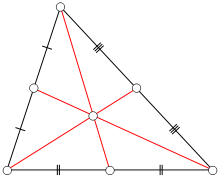Centroid of a triangle

In mathematics and physics, the centroid or geometric center of a plane figure is the arithmetic mean ("average") position of all the points in the shape. The definition extends to any object in n-dimensional space: its centroid is the mean position of all the points in all of the coordinate directions. Informally, it is the point at which an infinitesimally thin cutout of the shape could be perfectly balanced on the tip of a pin (assuming uniform density and a uniform gravitational field).

While in geometry the term "barycenter" is a synonym for "centroid", in astrophysics and astronomy, barycenter is the center of mass of two or more bodies which are orbiting each other. In physics, the center of mass is the arithmetic mean of all points weighted by the local density or specific weight. If a physical object has uniform density, then its center of mass is the same as the centroid of its shape.

In geography, the centroid of a radial projection of a region of the Earth's surface to sea level is known as the region's geographical center.

## Properties

The geometric centroid of a convex object always lies in the object. A non-convex object might have a centroid that is outside the figure itself. The centroid of a ring or a bowl, for example, lies in the object's central void.

If the centroid is defined, it is a fixed point of all isometries in its symmetry group. In particular, the geometric centroid of an object lies in the intersection of all its hyperplanes of symmetry. The centroid of many figures (regular polygon, regular polyhedron, cylinder, rectangle, rhombus, circle, sphere, ellipse, ellipsoid, superellipse, superellipsoid, etc.) can be determined by this principle alone.

In particular, the centroid of a parallelogram is the meeting point of its two diagonals. This is not true for other quadrilaterals.

For the same reason, the centroid of an object with translational symmetry is undefined (or lies outside the enclosing space), because a translation has no fixed point.

## Triangle centroid

The centroid of a triangle is the intersection of the three medians of the triangle (each median connecting a vertex with the midpoint of the opposite side). It lies on the triangle's Euler line, which also goes through various other key points including the orthocenter and the circumcenter.

Any of the three medians through the centroid divides the triangle's area in half. This is not true for other lines through the centroid; the greatest departure from the equal-area division occurs when a line through the centroid is parallel to a side of the triangle, creating a smaller triangle and a trapezoid; in this case the trapezoid's area is 5/9 that of the original triangle.

Let P be any point in the plane of a triangle with vertices A, B, and C and centroid G. Then the sum of the squared distances of P from the three vertices exceeds the sum of the squared distances of the centroid G from the vertices by three times the squared distance between P and G:The sum of the squares of the triangle's sides equals three times the sum of the squared distances of the centroid from the vertices:A triangle's centroid is the point that maximizes the product of the directed distances of a point from the triangle's sidelines.

For other properties of a triangle's centroid, see below.

## Locating the centroid

### Plumb line method

The centroid of a uniform planar lamina, such as (a) below, may be determined, experimentally, by using a plumbline and a pin to find the center of mass of a thin body of uniform density having the same shape. The body is held by the pin inserted at a point near the body's perimeter, in such a way that it can freely rotate around the pin; and the plumb line is dropped from the pin (b). The position of the plumbline is traced on the body. The experiment is repeated with the pin inserted at a different point of the object. The intersection of the two lines is the centroid of the figure (c).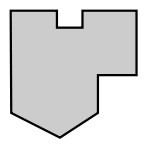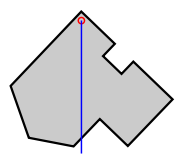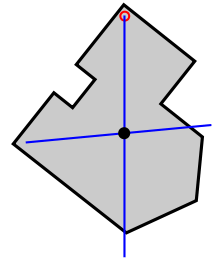(a) (b) (c)

This method can be extended (in theory) to concave shapes where the centroid lies outside the shape, and to solids (of uniform density), but the positions of the plumb lines need to be recorded by means other than drawing.

### Balancing method

For convex two-dimensional shapes, the centroid can be found by balancing the shape on a smaller shape, such as the top of a narrow cylinder. The centroid occurs somewhere within the range of contact between the two shapes. In principle, progressively narrower cylinders can be used to find the centroid to arbitrary precision. In practice air currents make this unfeasible. However, by marking the overlap range from multiple balances, one can achieve a considerable level of accuracy.

### Of a finite set of points

The centroid of a finite set ofpointsinis.

This point minimizes the sum of squared Euclidean distances between itself and each point in the set.

### By geometric decomposition

The centroid of a plane figurecan be computed by dividing it into a finite number of simpler figures, computing the centroidand areaof each part, and then computingHoles in the figure, overlaps between the parts, or parts that extend outside the figure can all be handled using negative areas. Namely, the measuresshould be taken with positive and negative signs in such a way that the sum of the signs offor all parts that enclose a given pointis 1 ifbelongs to, and 0 otherwise.

For example, the figure below (a) is easily divided into a square and a triangle, both with positive area; and a circular hole, with negative area (b).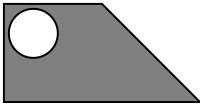(a) 2D Object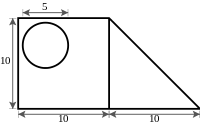(b) Object described using simpler elements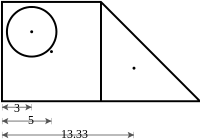(c) Centroids of elements of the object

The centroid of each part can be found in any list of centroids of simple shapes (c). Then the centroid of the figure is the weighted average of the three points. The horizontal position of the centroid, from the left edge of the figure isThe vertical position of the centroid is found in the same way.

The same formula holds for any three-dimensional objects, except that eachshould be the volume of, rather than its area. It also holds for any subset of, for any dimension, with the areas replaced by the-dimensional measures of the parts.

### By integral formula

The centroid of a subset X ofcan also be computed by the integralwhere the integrals are taken over the whole space, and g is the characteristic function of the subset, which is 1 inside X and 0 outside it. Note that the denominator is simply the measure of the set X. This formula cannot be applied if the set X has zero measure, or if either integral diverges.

Another formula for the centroid iswhere Ck is the kth coordinate of C, and Sk(z) is the measure of the intersection of X with the hyperplane defined by the equation xk = z. Again, the denominator is simply the measure of X.

For a plane figure, in particular, the barycenter coordinates arewhere A is the area of the figure X; Sy(x) is the length of the intersection of X with the vertical line at abscissa x; and Sx(y) is the analogous quantity for the swapped axes.

#### Bounded region

The centroidof a region bounded by the graphs of the continuous functionsandsuch thaton the interval,, is given bywhereis the area of the region (given by).

### Of an L-shaped object

This is a method of determining the centroid of an L-shaped object.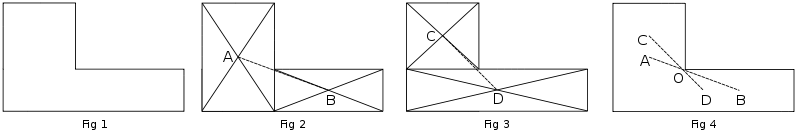1. Divide the shape into two rectangles, as shown in fig 2. Find the centroids of these two rectangles by drawing the diagonals. Draw a line joining the centroids. The centroid of the shape must lie on this line AB.
2. Divide the shape into two other rectangles, as shown in fig 3. Find the centroids of these two rectangles by drawing the diagonals. Draw a line joining the centroids. The centroid of the L-shape must lie on this line CD.
3. As the centroid of the shape must lie along AB and also along CD, it is obvious that it is at the intersection of these two lines, at O. The point O might not lie inside the L-shaped object.

### Of triangle and tetrahedron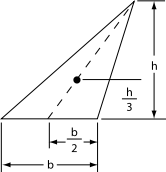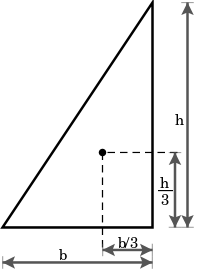The centroid of a triangle is the point of intersection of its medians (the lines joining each vertex with the midpoint of the opposite side). The centroid divides each of the medians in the ratio 2:1, which is to say it is located ⅓ of the distance from each side to the opposite vertex (see figures at right). Its Cartesian coordinates are the means of the coordinates of the three vertices. That is, if the three vertices areandthen the centroid (denoted C here but most commonly denoted G in triangle geometry) isThe centroid is therefore atin barycentric coordinates.

In trilinear coordinates the centroid can be expressed in any of these equivalent ways in terms of the side lengths a, b, c and vertex angles L, M, N:The centroid is also the physical center of mass if the triangle is made from a uniform sheet of material; or if all the mass is concentrated at the three vertices, and evenly divided among them. On the other hand, if the mass is distributed along the triangle's perimeter, with uniform linear density, then the center of mass lies at the Spieker center (the incenter of the medial triangle), which does not (in general) coincide with the geometric centroid of the full triangle.

The area of the triangle is 1.5 times the length of any side times the perpendicular distance from the side to the centroid.

A triangle's centroid lies on its Euler line between its orthocenter H and its circumcenter O, exactly twice as close to the latter as to the former:In addition, for the incenter I and nine-point center N, we haveSimilar results hold for a tetrahedron: its centroid is the intersection of all line segments that connect each vertex to the centroid of the opposite face. These line segments are divided by the centroid in the ratio 3:1. The result generalizes to any n-dimensional simplex in the obvious way. If the set of vertices of a simplex is, then considering the vertices as vectors, the centroid isThe geometric centroid coincides with the center of mass if the mass is uniformly distributed over the whole simplex, or concentrated at the vertices as n equal masses.

The isogonal conjugate of a triangle's centroid is its symmedian point.

### Centroid of polygon

The centroid of a non-self-intersecting closed polygon defined by n vertices (x0,y0), (x1,y1), ..., (xn1,yn1) is the point (Cx, Cy), whereand where A is the polygon's signed area,.

In these formulas, the vertices are assumed to be numbered in order of their occurrence along the polygon's perimeter. Furthermore, the vertex ( xn, yn ) is assumed to be the same as ( x0, y0 ), meaning i + 1 on the last case must loop around to i = 0. Note that if the points are numbered in clockwise order the area A, computed as above, will have a negative sign; but the centroid coordinates will be correct even in this case.

### Centroid of cone or pyramid

The centroid of a cone or pyramid is located on the line segment that connects the apex to the centroid of the base. For a solid cone or pyramid, the centroid is 1/4 the distance from the base to the apex. For a cone or pyramid that is just a shell (hollow) with no base, the centroid is 1/3 the distance from the base plane to the apex.

## Tetrahedron

A tetrahedron is an object in three-dimensional space having four triangles as its faces. A line segment joining a vertex of a tetrahedron with the centroid of the opposite face is called a median, and a line segment joining the midpoints of two opposite edges is called a bimedian. Hence there are four medians and three bimedians. These seven line segments are all concurrent at the centroid of the tetrahedron. The centroid of a tetrahedron is the midpoint between its Monge point and circumcenter. These points define the Euler line of the tetrahedron that is analogous to the Euler line of a triangle.

## Notes

1. Altshiller-Court (1925, p. 101)
2. Kay (1969, pp. 18,189,225–226)
3. Bottomley, Henry. "Medians and Area Bisectors of a Triangle". Retrieved 27 September 2013.
4. Altshiller-Court (1925, pp. 70–71)
5. Altshiller-Court (1925, pp. 70–71)
6. Clark Kimberling, "Trilinear distance inequalities for the symmedian point, the centroid, and other triangle centers", Forum Geometricorum, 10 (2010), 135--139. http://forumgeom.fau.edu/FG2010volume10/FG201015index.html
7. Protter & Morrey, Jr. (1970, p. 520)
8. Protter & Morrey, Jr. (1970, p. 526)
9. Protter & Morrey, Jr. (1970, p. 526)
10. Protter & Morrey, Jr. (1970, p. 527)
11. Protter & Morrey, Jr. (1970, p. 528)
12. Larson (1998, pp. 458–460)
13. Clark Kimberling's Encyclopedia of Triangles "Archived copy". Archived from the original on 2012-04-19. Retrieved 2012-06-02.
14. Johnson (2007, p. 173)
15. Bourke (July 1997)
16. Leung, Kam-tim; and Suen, Suk-nam; "Vectors, matrices and geometry", Hong Kong University Press, 1994, pp. 53–54